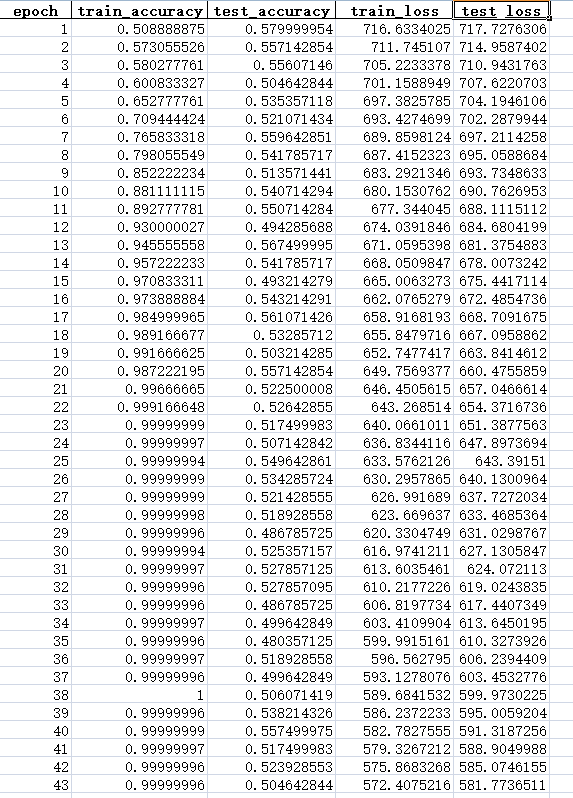tensorflow模型，4层cnn1个回答FCN语义分割脑肿瘤，训练精度很高，测试精度很低

tensorflow CNN训练图片分类的时候，模型训练不出来，准确率0.1（分了十类），模型失效，是什么原因？

``` def compute_accuracy(v_xs, v_ys): global prediction y_pre = sess.run(prediction, feed_dict={xs: v_xs, keep_prob: 1}) correct_prediction = tf.equal(tf.argmax(y_pre,1), tf.argmax(v_ys,1)) accuracy = tf.reduce_mean(tf.cast(correct_prediction, tf.float32)) result = sess.run(accuracy, feed_dict={xs: v_xs, ys: v_ys, keep_prob: 1}) return result def weight_variable(shape): initial = tf.truncated_normal(shape, stddev=0.1) return tf.Variable(initial) def bias_variable(shape): initial = tf.constant(0.1, shape=shape) return tf.Variable(initial) def conv2d(x, W): # stride [1, x_movement, y_movement, 1] # Must have strides = strides = 1 return tf.nn.conv2d(x, W, strides=[1, 1, 1, 1], padding='SAME') def max_pool_2x2(x): # stride [1, x_movement, y_movement, 1] return tf.nn.max_pool(x, ksize=[1,4,4,1], strides=[1,4,4,1], padding='SAME') # define placeholder for inputs to network xs = tf.placeholder(tf.float32, [None, 65536])/255. # 256x256 ys = tf.placeholder(tf.float32, [None, 10]) keep_prob = tf.placeholder(tf.float32) x_image = tf.reshape(xs, [-1, 256, 256, 1]) # print(x_image.shape) # [n_samples, 28,28,1] ## conv1 layer ## W_conv1 = weight_variable([3,3, 1,64]) # patch 5x5, in size 1, out size 32 b_conv1 = bias_variable() h_conv1 = tf.nn.elu(conv2d(x_image, W_conv1) + b_conv1) # output size 28x28x32 h_pool1 = tf.nn.max_pool(h_conv1, ksize=[1,2,2,1], strides=[1,2,2,1], padding='SAME') # output size 14x14x32 ## conv2 layer ## W_conv2 = weight_variable([3,3, 64, 128]) # patch 5x5, in size 32, out size 64 b_conv2 = bias_variable() h_conv2 = tf.nn.elu(conv2d(h_pool1, W_conv2) + b_conv2) # output size 14x14x64 h_pool2 = max_pool_2x2(h_conv2) # output size 7x7x64 ## conv3 layer ## W_conv3 = weight_variable([3,3, 128, 256]) # patch 5x5, in size 32, out size 64 b_conv3 = bias_variable() h_conv3 = tf.nn.elu(conv2d(h_pool2, W_conv3) + b_conv3) # output size 14x14x64 h_pool3 = max_pool_2x2(h_conv3) ## conv4 layer ## W_conv4 = weight_variable([3,3, 256, 512]) # patch 5x5, in size 32, out size 64 b_conv4 = bias_variable() h_conv4 = tf.nn.elu(conv2d(h_pool3, W_conv4) + b_conv4) # output size 14x14x64 h_pool4 = max_pool_2x2(h_conv4) # ## conv5 layer ## # W_conv5 = weight_variable([3,3, 512, 512]) # patch 5x5, in size 32, out size 64 # b_conv5 = bias_variable() # h_conv5 = tf.nn.relu(conv2d(h_pool3, W_conv4) + b_conv4) # output size 14x14x64 # h_pool5 = max_pool_2x2(h_conv4) ## fc1 layer ## W_fc1 = weight_variable([2*2*512, 128]) b_fc1 = bias_variable() # [n_samples, 7, 7, 64] ->> [n_samples, 7*7*64] h_pool4_flat = tf.reshape(h_pool4, [-1, 2*2*512]) h_fc1 = tf.nn.elu(tf.matmul(h_pool4_flat, W_fc1) + b_fc1) h_fc1_drop = tf.nn.dropout(h_fc1, keep_prob) ## fc2 layer ## W_fc2 = weight_variable([128, 10]) b_fc2 = bias_variable() prediction = tf.nn.softmax(tf.matmul(h_fc1_drop, W_fc2) + b_fc2) # 定义优化器和训练op loss = tf.reduce_mean(tf.nn.softmax_cross_entropy_with_logits(labels=ys, logits=prediction)) train_step = tf.train.RMSPropOptimizer((1e-3)).minimize(loss) correct_prediction = tf.equal(tf.argmax(prediction, 1), tf.argmax(ys, 1)) accuracy = tf.reduce_mean(tf.cast(correct_prediction, tf.float32)) # 用于保存和载入模型 saver = tf.train.Saver() def int2onehot(train_batch_ys): num_labels = train_batch_ys.shape num_classes=10 index_offset = np.arange(num_labels) * num_classes labels_one_hot = np.zeros((num_labels, num_classes),dtype=np.float32) labels_one_hot.flat[index_offset + train_batch_ys.ravel()] = 1 return labels_one_hot train_label_lists, train_data_lists, train_fname_lists = read_tfrecords(train_tfrecord_file) iterations = 100 with tf.Session() as sess: sess.run(tf.global_variables_initializer()) # 执行训练迭代 for it in range(iterations): # 这里的关键是要把输入数组转为np.array for i in range(200): train_label_list = train_label_lists[i] train_data_list= train_data_lists[i] train_name_list = train_fname_lists[i] #print("shape of train_data_list: {}\tshape of train_label_list: {}".format(train_data_list.shape, train_label_list.shape)) #print('该批文件名：',train_name_list) print('该批标签：',train_label_list) # 计算有多少类图片 #num_classes = len(set(train_label_list)) #print("num_classes:",num_classes) train_batch_xs = train_data_list train_batch_xs = np.reshape(train_batch_xs, (-1, 65536)) train_batch_ys = train_label_list train_batch_ys = int2onehot(train_batch_ys) #print('第'+str(i)+'批-----------') print("连接层1之后----------------------------------------") for i in range(80): print("元素"+str(i)+"：",sess.run(tf.reduce_mean(sess.run(h_fc1_drop,feed_dict={xs: train_batch_xs, ys: train_batch_ys, keep_prob: 0.5})[i].shape))) print("元素"+str(i)+"：",sess.run(h_fc1_drop,feed_dict={xs: train_batch_xs, ys: train_batch_ys, keep_prob: 0.5})[i]) print("连接层2之后----------------------------------------") for i in range(80): print("元素"+str(i)+"：",sess.run(tf.reduce_mean(sess.run(prediction,feed_dict={xs: train_batch_xs, ys: train_batch_ys, keep_prob: 0.5})[i].shape))) print("元素"+str(i)+"：",sess.run(prediction,feed_dict={xs: train_batch_xs, ys: train_batch_ys, keep_prob: 0.5})[i]) #loss.run(feed_dict={xs: train_batch_xs, ys: train_batch_ys, keep_prob: 0.5}) train_step.run(feed_dict={xs: train_batch_xs, ys: train_batch_ys, keep_prob: 0.5}) time.sleep(7) # 每完成五次迭代，判断准确度是否已达到100%，达到则退出迭代循环 iterate_accuracy = 0 if it%5 == 0: iterate_accuracy = accuracy.eval(feed_dict={xs: train_batch_xs, ys: train_batch_ys, keep_prob: 0.5}) print ('iteration %d: accuracy %s' % (it, iterate_accuracy)) if iterate_accuracy >= 1: break; print ('完成训练!') ```

TensorFlow2.0训练模型时，指标不收敛一直上升到1

softmax分类器，分类概率都是nan是怎么回事？

python如何自定义权重损失函数？

Java 最常见的 200+ 面试题：面试必备

java jdk 8 帮助文档 中文 文档 chm 谷歌翻译

JDK1.8 API 中文谷歌翻译版 java帮助文档 JDK API java 帮助文档 谷歌翻译 JDK1.8 API 中文 谷歌翻译版 java帮助文档 Java最新帮助文档 本帮助文档是使用谷

csma/ca和csma/cd的matlab仿真源代码带有详细的注释

csma/ca和csma/cd的matlab仿真源代码带有详细的注释，载波侦听，随意设置节点数，带有炫酷的图形展示效果。

Java基础知识面试题（2020最新版）

pokemmo的资源

pokemmo必须的4个rom 分别为绿宝石 火红 心金 黑白 还有汉化补丁 资源不错哦 记得下载

Java8零基础入门视频教程

Python数据清洗实战入门

linux下利用/proc进行进程树的打印

MySQL数据库面试题（2020最新版）

HoloLens2开发入门教程

150讲轻松搞定Python网络爬虫

【为什么学爬虫？】 &nbsp; &nbsp; &nbsp; &nbsp;1、爬虫入手容易，但是深入较难，如何写出高效率的爬虫，如何写出灵活性高可扩展的爬虫都是一项技术活。另外在爬虫过程中，经常容易遇到被反爬虫，比如字体反爬、IP识别、验证码等，如何层层攻克难点拿到想要的数据，这门课程，你都能学到！ &nbsp; &nbsp; &nbsp; &nbsp;2、如果是作为一个其他行业的开发者，比如app开发，web开发，学习爬虫能让你加强对技术的认知，能够开发出更加安全的软件和网站 【课程设计】 一个完整的爬虫程序，无论大小，总体来说可以分成三个步骤，分别是： 网络请求：模拟浏览器的行为从网上抓取数据。 数据解析：将请求下来的数据进行过滤，提取我们想要的数据。 数据存储：将提取到的数据存储到硬盘或者内存中。比如用mysql数据库或者redis等。 那么本课程也是按照这几个步骤循序渐进的进行讲解，带领学生完整的掌握每个步骤的技术。另外，因为爬虫的多样性，在爬取的过程中可能会发生被反爬、效率低下等。因此我们又增加了两个章节用来提高爬虫程序的灵活性，分别是： 爬虫进阶：包括IP代理，多线程爬虫，图形验证码识别、JS加密解密、动态网页爬虫、字体反爬识别等。 Scrapy和分布式爬虫：Scrapy框架、Scrapy-redis组件、分布式爬虫等。 通过爬虫进阶的知识点我们能应付大量的反爬网站，而Scrapy框架作为一个专业的爬虫框架，使用他可以快速提高我们编写爬虫程序的效率和速度。另外如果一台机器不能满足你的需求，我们可以用分布式爬虫让多台机器帮助你快速爬取数据。 &nbsp; 从基础爬虫到商业化应用爬虫，本套课程满足您的所有需求！ 【课程服务】 专属付费社群+每周三讨论会+1v1答疑

MySQL 8.0.19安装教程(windows 64位)

Python数据挖掘简易入门

&nbsp; &nbsp; &nbsp; &nbsp; 本课程为Python数据挖掘方向的入门课程，课程主要以真实数据为基础，详细介绍数据挖掘入门的流程和使用Python实现pandas与numpy在数据挖掘方向的运用，并深入学习如何运用scikit-learn调用常用的数据挖掘算法解决数据挖掘问题，为进一步深入学习数据挖掘打下扎实的基础。

Ubuntu18.04安装教程

Ubuntu18.04.1安装一、准备工作1.下载Ubuntu18.04.1 LTS2.制作U盘启动盘3.准备 Ubuntu18.04.1 的硬盘空间二、安装Ubuntu18.04.1三、安装后的一些工作1.安装输入法2.更换软件源四、双系统如何卸载Ubuntu18.04.1新的改变功能快捷键合理的创建标题，有助于目录的生成如何改变文本的样式插入链接与图片如何插入一段漂亮的代码片生成一个适合你的列...

sql语句 异常 Err] 1064 - You have an error in your SQL syntax; check the manual that corresponds to your

Windows版YOLOv4目标检测实战：训练自己的数据集

Spring Boot -01- 快速入门篇（图文教程）

Spring Boot -01- 快速入门篇 今天开始不断整理 Spring Boot 2.0 版本学习笔记，大家可以在博客看到我的笔记，然后大家想看视频课程也可以到【慕课网】手机 app，去找【Spring Boot 2.0 深度实践】的课程，令人开心的是，课程完全免费！ 什么是 Spring Boot？ Spring Boot 是由 Pivotal 团队提供的全新框架。Spring Boot...

C/C++学习指南全套教程

C/C++学习的全套教程，从基本语法，基本原理，到界面开发、网络开发、Linux开发、安全算法，应用尽用。由毕业于清华大学的业内人士执课，为C/C++编程爱好者的教程。

winfrom中嵌套html，跟html的交互

winfrom中嵌套html，跟html的交互,源码就在里面一看就懂，很简单